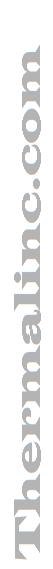Thermalinc.com CalculatorHeat Basics Methods of Heat Transfer Glossary of Terms Why This Page Over the years, some basic questions keep coming up over and over again. Our people ask a lot of questions when sizing a heater system. The answers to these questions determine the effectiveness of the end product. Many times cost ends up as the most important determinant and without real knowledge of the all the factors involved it is impossible to arrive at a good thermal system. Note:   This is designed to cover some basic subjects in as non-technical language as possible. Therefore, let's start with some of the very basic principles of heat. How heat works Heat is derived from the motion of molecules, the faster the molecules move the hotter the object becomes. Heat always flows from a substance with a higher temperature to a substance with a lower temperature. That is to say, that heat is removed from one substance and transferred to the cooler surface. The energy is transferred to the substance with the lesser energy. This is important because if we can maintain the temperature surrounding a substance with an equal temperature we can maintain the temperature. But, if the surrounding temperature is cooler we will remove energy or "heat" from the substance to the surrounding area. Temperature can be thought of as two separate variables. Intensity, and Quantity Intensity is the temperature and can be measured is F or C for this discussion. Quantity is the amount of energy required to raise the temperature and can be measured in "British Thermal Units". The BTU is the amount of heat required to heat 1 pound of water 1 degree Fahrenheit in 1 hour. There are at least three (3) categories of heat; Specific heat, Sensible heat, and Latent heat. Specific heat If a BTU is the amount of heat required to heat 1 pound of water 1 degree Fahrenheit, we can establish a relationship with other materials. One(1) pound of steel, 1 pound of air, 1 pound of any substance as to how many BTU's are required to raise the temperature 1 degree Fahrenheit. As it works out it takes less heat (or BTU's) to heat everything than water. This relationship is the specific heat of a substance.. The specific heat of water is 1 or it takes 1 BTU to raise water 1 degree Fahrenheit. The specific heat of mild steel is .122 therefore, it takes .122 BTU's to raise steel 1 degree Fahrenheit. If we know the specific heat of a material, the weight of the material, the desired temperature rise and the amount of time we would like to raise the temperature to we can arrive at a simple formula. BTU' Required = Desired temperature rise * Specific heat * weight of the material / time to temperature in hours. Sensible heat Is simply the amount of heat that can be sensed. Therefore, sensible heat is the heat that effects a change on the temperature or intensity of a substance. If heat is applied and the temperature changes the sensible heat is the amount of heat that was used to create the change. Latent heat Latent heat is the heat that is unseen. Although the heat is being transferred into the substance the temperature of the material does not change. This is the heat required to change the state of a material. If water is raised to 212°s Fahrenheit the amount of heat required to raise the water each degree is the same (1 BTU ). But, once the temperature reaches boiling point the temperature does not continue to rise. During this time you can see the effects of latent heat, not with the water temperature, but in the generation of steam. This same principle can be seen in any material, Ice to Water, Steel to molten steel, many materials from liquid to gas.

We've seen your application. Call Us for application Assistance